##### Circuit Analysis For Dummies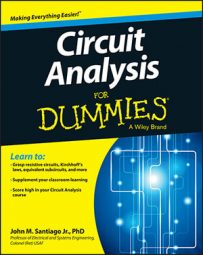Mesh-current analysis (loop-current analysis) can help reduce the number of equations you must solve during circuit analysis. Mesh-current analysis is simply Kircholff’s voltage law adapted for circuits that have many devices connected in multiple loops.

## Analyze two-mesh circuits

This section walks you through mesh-current analysis when you have two equations, one for Mesh A and one for Mesh B. In the sample circuit shown here, both meshes have a clockwise current.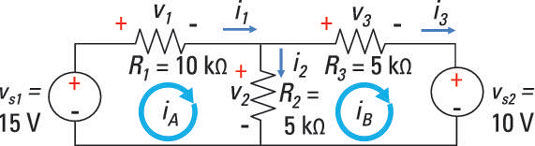The next step is to apply KVL to Mesh A and B to arrive at the following mesh equations: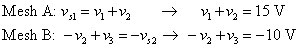Next, write the device currents in terms of mesh currents. Then express the device currents in terms of the mesh currents using Ohm’s law: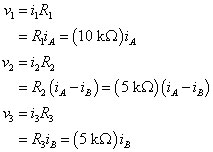Now you can substitute the preceding voltage values into the KVL equations you found earlier: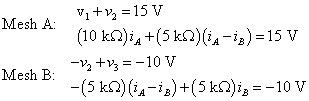When you rearrange the preceding equations to put them in standard form, you get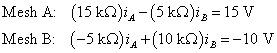Converting these mesh equations into matrix form results in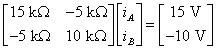The preceding equation has the form Ax = b, where matrix A is the coefficients of resistors, x is a vector of unknown mesh currents, and b is a vector of independent voltage sources.

You can use your graphing calculator or matrix software to give you the mesh currents: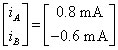With these calculated mesh currents, you can find the device currents:

i1 = iA = 0.8 mA
i2 = iAiB = 0.8 mA – (–0.6 mA) = 1.4 mA

To complete the analysis, plug the device currents and resistances into the Ohm’s law equations. You find the following device voltages:

v1 = i1R1 = (0.8 mA)(10 kΩ) = 8 V
v2 = i2R2 = (1.5 mA)(5 kΩ) = 7 V
v3 = i3R3 = (–0.6 mA)(5 kΩ) = –3 V

The preceding device voltages make sense because they satisfy KVL for each mesh.

## Analyze circuits with three or more meshes

You can apply mesh-current analysis when dealing with circuits that have three or more meshes. The process is the same as for circuits with only two mesh currents. Consider this sample circuit, which shows voltages and currents for each of the devices as well as the mesh currents iA, iB, and iC. Notice that all the mesh currents flow clockwise.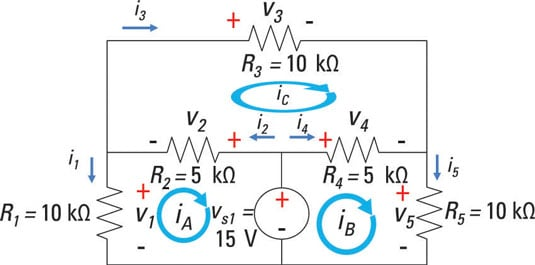The KVL equations for Meshes A, B, and C are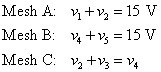Now express the device currents in terms of mesh currents. Then apply Ohm’s law to get the element voltages in terms of the mesh currents: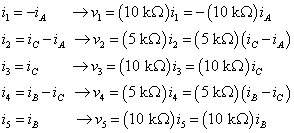When you substitute the preceding device voltages into the KVL equations found earlier, you wind up with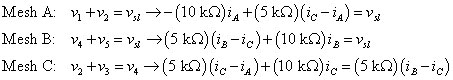Rearrange the equations to put them in standard form. You can insert some zeros as placeholder terms to help you set up the matrices in the next step: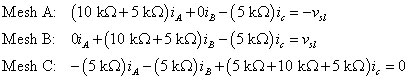And you can translate these standard-form equations into matrix form to get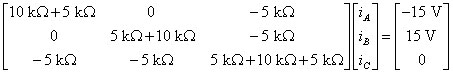Simplify the elements in the resistor matrix: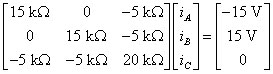Notice that in the resistor matrix, the main-diagonal values are all positive, the off-diagonal values are all negative or zero, and the off-diagonal values are symmetric. For a circuit with an independent source, that symmetry with respect to the main diagonal is a good sign that you’ve set up the problem correctly.

You can use your graphing calculator or matrix software to find the mesh currents: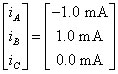The current iC = 0 makes sense due to the circuit symmetry. With these calculated values for mesh currents, you find the following device currents: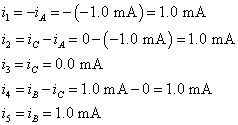To complete the analysis, calculate the device voltages using Ohm’s law, relating the device currents and voltages: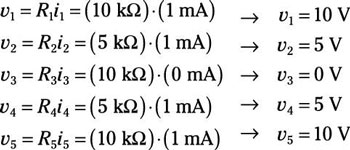The preceding results make sense because they satisfy the KVL equations for the three meshes.